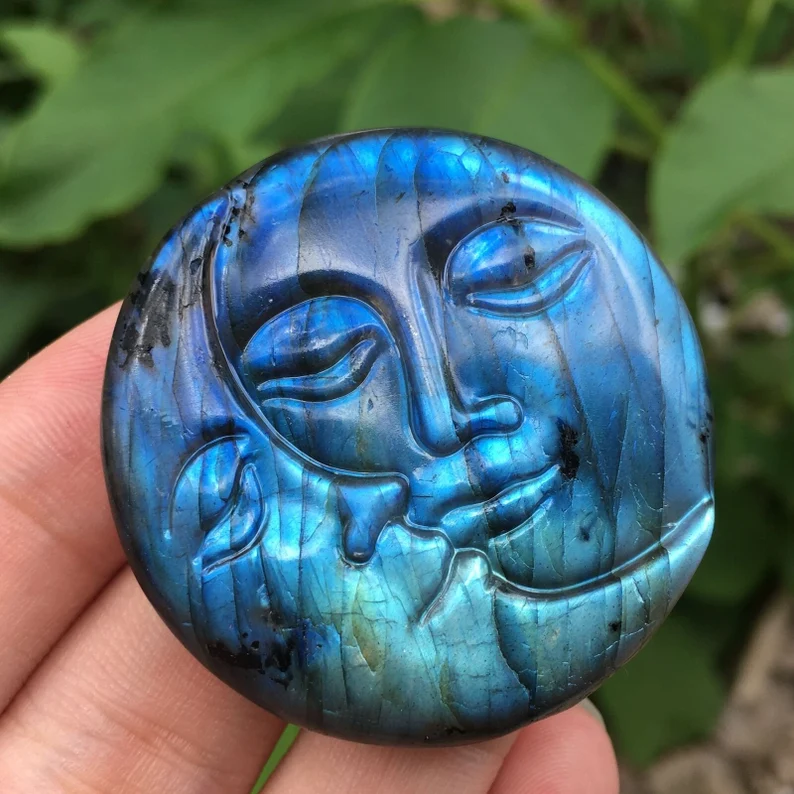• English
• United States（USD \$）
• \$ USD
• £ GBP
• EUR
• A\$ AUD
• INR
• ¥ JPY
• د.إ AED
• Af AFN
• L ALL
• Դ AMD
• ƒ ANG
• Kz AOA
• \$ ARS
• ƒ AWG
• ман AZN
• КМ BAM
• BBD BBD
• BDT
• лв BGN
• BIF
• BD\$ BMD
• B\$ BND
• Bs. BOB
• BOV BOV
• R\$ BRL
• BSD BSD
• Nu. BTN
• P BWP
• Br BYN
• Be BZD
• CDF
• CHF
• CLP CLP
• ¥ CNY
• \$ COP
• CRC
• \$ CUC
• \$ CUP
• \$ CVE
• CZK
• DJF
• kr DKK
• \$ DOP
• د.ج DZD
• EGP
• Nfk ERN
• Br ETB
• FJ\$ FJD
• FKP FKP
• GEL
• GHS
• £ GIP
• D GMD
• GNF
• Q GTQ
• \$ GYD
• HK\$ HKD
• L HNL
• Kn HRK
• G HTG
• Ft HUF
• Rp IDR
• ILS
• IRR
• Kr ISK
• \$ JMD
• Sh KES
• С̲ KGS
• KHR
• Fr KMF
• KPW
• KRW
• \$ KYD
• KZT
• LAK
• ل.ل LBP
• Rs LKR
• L\$ LRD
• L LSL
• L MDL
• ден MKD
• K MMK
• MNT
• P MOP
• MUR
• ރ. MVR
• MK MWK
• \$ MXN
• RM MYR
• MTn MZN
• NGN
• C\$ NIO
• kr NOK
• NPR
• \$ NZD
• B/. PAB
• S/ PEN
• K PGK
• PHP
• PKR
• PLN
• PYG
• ر.ق QAR
• L RON
• din RSD
• р. RUB
• RWF
• ر.س SAR
• Si\$ SBD
• SCR
• ج.س. SDG
• kr SEK
• S\$ SGD
• £ SHP
• Le SLL
• Sh SOS
• \$ SRD
• SDP SSP
• Db STN
• ل.س SYP
• L SZL
• ฿ THB
• ЅМ TJS
• m TMT
• T\$ TOP
• TRY
• TTD TTD
• NT\$ TWD
• Sh TZS
• UAH
• Sh UGX
• \$ UYU
• лв UZS
• Bs.S. VES
• VND
• Vt VUV
• T WST
• XAF
• \$ XCD
• Fr XOF
• XPF
• YER
• R ZAR
• ZK ZMW
• دينار KWD
• ر.ع. OMR
• .د.ب BHD

No relevant currency found

Free Shipping on orders over \$49.99!
Buy 3 Items Get Extra 10% OFF Again\$3299
\$000
30-Day returns
Carbon neutral
Secure payments
Quantity
Only 1999 left

FAST RETURN & REFUND SERVICE
24H ONLINE SERVICE

Description